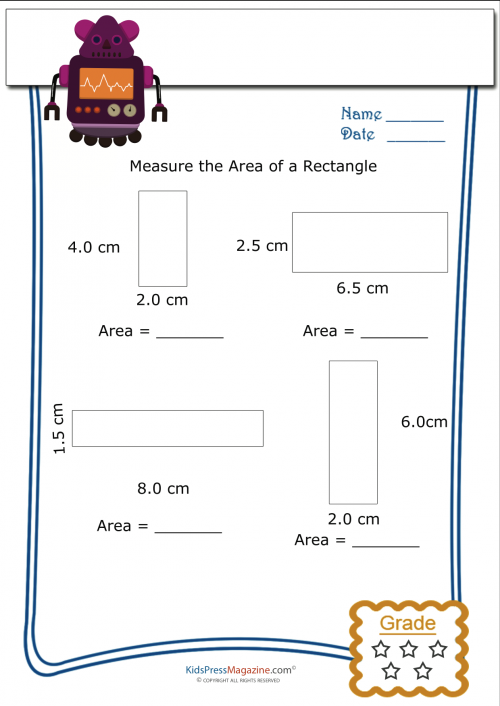# Measuring Area Worksheet – Rectangle 4

Between third and fifth grade your child will begin to learn algebra and geometry. Learning one tends to help the learning of the other as algebra gives the equations used in geometry and geometry gives a visual of how algebra equations work. Use this free geometry worksheet to help your child understand how to calculate the area of a rectangle!